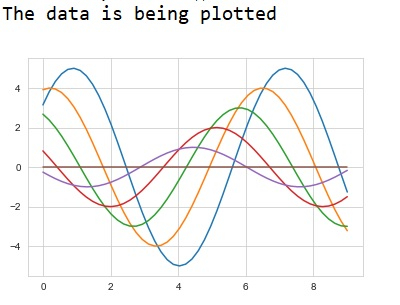# How can data be represented visually using ‚Äòseaborn‚Äô library in Python?

Machine learning deals with creating models from data, and generalizing on never before seen data. The data provided to a machine learning model as input should be such that it should be understood by the system properly, so that it can interpret the data and produce results.

Seaborn is a library that helps in visualizing data. It comes with customized themes and a high-level interface. This interface helps in customizing and controlling the kind of data and how it behaves when certain filters are applied to it.

Seaborn library contains an interface called ‘set_Style()’ that helps work with different styles. The theme of the plot can be set using the above mentioned function.

Let us try to visualize a simple dataset using Seaborn in Python −

## Example

import numpy as np
from matplotlib import pyplot as plt
def sine_plot(flip=1):
x = np.linspace(0, 9, 50)
for i in range(1, 7):
plt.plot(x, np.sin(x + i * .68) * (6 - i) * flip)
import seaborn as sb
sb.set_style("whitegrid")
print("The data is being plotted ")
sine_plot()
plt.show()

## Output## Explanation

• The required packages are imported.
• The input data is generated using the user defined function named ‘sine_plot’.
• The set_style function is used to set the type of plot.
• This data is specified to be plotted using seaborn library
• This visual data is displayed on the console.